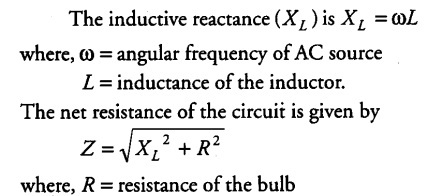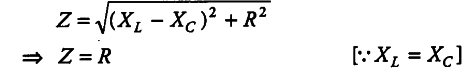# An inductor L of inductance XL is connected in series

An inductor L of inductance XL is connected in series with a bulb Band an AC source. How would brightness of the bulb change when
(i) number of turns in the inductor is reduced
(ii) an iron rod is inserted in the inductor and
(iii) a capacitor of reactance Xc = XL is inserted in series in the circuit. Justify your answer in each case.(i) We know that if the number of turns in the inductor
decreases, then inductance L decreases. So, the net resistance of the circuit decreases and hence, the current through the circuit increases, increasing the brightness of the bulb.
(ii) If the soft iron rod is inserted in the inductor, then the inductance L increases. Therefore, the current through the bulb will decrease, decreasing the brightness of the bulb.
(iii) If the capacitor of reactance Xc = XL is connected in series with the circuit, thenThis is a case of resonance. In this case, the maximum current will flow through the circuit. Hence, the brightness of the bulb will increase.